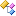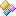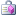﻿DHI.Projections Namespace

# DHI.Projections Namespace

### Class library documentation for the DHI.Projections.

The DHI.Projections library handles conversion between various map projections and coordinate systems.

The important classes in the DHI.Projections namespace are:

• MapProjection: A map projection handles conversion from geographical coordinates (lon, lat) to projection coordintes (east, north) and to Euclidean 3D datum coordinates with origin in the center of the earch (center of the ellipsoid).
• Cartography: A cartography object handles a map projection and a local grid that can be translated and rotated relative to the projected coordinate system.
• Reprojector: The Reprojector class handles conversion from a source map projection to a target map projection. The conversion can be performed with or without height. The conversion automatically handles datum (ellipsoid) conversions, and can also perform datum shifts.
• GridReprojector: Class for reprojecting a source grid in one coordinate system to a target grid in another coordinate system.Classes
ClassDescriptionCartography
A cartography object handles a map projection and a local grid that can be translated and rotated relative to the projected coordinate system.

There are 3 levels of coordinates: Geographical coordinates (longitude, latitude) in degrees, projection coordinates (easting, northing), and local/model/user grid coordinates (x,y).

The ProjectionString defines the mapping from geographical coordinates to projection coordinates.

The LatOrigin, LonOrigin and Orientation defines the origin and the orientation of the local grid coordinates. It defines how the local grid coordinate system is translated and rotated. This kind of local grid coordinate system is used for e.g. a 2D grid in a dfs2 file.

See Orientation for the definition of the orientation. The local grid are rotated around its origin.

If LonOrigin is zero, and LatOrigin and ProjectionOrigin(String, Double, Double) matches the origin of the projection coordinate system (from MapProjection), then projection coordinates equals local grid coordinates. Example: UTM-31 has projection origin at (lon,lat) = (3,0).Function2DInterp
Class for interpolating double values from a function in 2D, i.e. a function on the form
`z = f(x,y)`

This class can be used when a function in 2D space is too expensive to evaluate. Function values are precalculated on a predefined 2D grid, and when calling Interpolate(Double, Double), values are interpolated from the values on the 2D grid.

Use the Resample function to increase the resolution of the 2D grid and thereby increase the accuracy if the interpolation.Function2DInterpBaseT
Base class (abstract) for interpolating values from a function in 2D.

This class can be used when a function in 2D space is too expensive to evaluate. Function values are precalculated on a predefined 2D grid, and when calling Interpolate(Double, Double), values are interpolated from the values on the 2D grid.

Use the Resample function to increase the resolution of the 2D grid and thereby increase the accuracy if the interpolation.Function2DInterpXyCoord
Class for interpolating coordinate values from a function in 2D, i.e. a function on the form
`(X,Y) = f(x,y)`

This class can be used when a function in 2D space is too expensive to evaluate. Function values are precalculated on a predefined 2D grid, and when calling Interpolate(Double, Double), values are interpolated from the values on the 2D grid.

Use the Resample function to increase the resolution of the 2D grid and thereby increase the accuracy if the interpolation.GridInterpolator
Class for interpolating values from a grid.

Interpolation is performed in two steps, first step is calculation of fractional indices and second step is interpolation based on fractional indices.

Fractional indices are calculated by calling one of the *2Jk methods. Values are interpolated by calling the Interpolate(Single, Double, Double, Single) method with the fractional indices.

Calculating fractional indices involves various map projection calculations and can be computational expensive. The fractional indices are meant to be stored in case they are to be reused.

By setting up reprojection, using one of the SetupReprojection methods, you can specify a target projection different from the grid projection. Then interpolation point coordinates provided in the *2Jk methods (as well Xy, Proj and Geo version) are assumed to be in that target map projection, and reprojection from the target projection to the grid projection will be performed before calculating fractional indices.GridReprojector
Class for reprojecting a source grid in one coordinate system to a target grid in another coordinate system.

Both source and target grids are regular in each their coordinate system. However, the source grid may not be a regular grid in the target coordinate system, so re-gridding and interpolation is necessary.

This class is intended for reuse, i.e. reprojection weights are stored and if executing the Convert(Single, Single, Single, Single) method more than once, the stored reprojection values are reused. This is for performance reasons, since the actual reprojection can be an expensive operation.GridReprojectorGridInfo
Grid info - defining origin, size and projection of gridInterpolatingConverter
Class for performing a conversion inside a user specified area. It utilizes bilinear interpolation on a grid of pre-converted values.

The assumption is that the original conversion is fairly expensive, and accuracy is not as important as performance.

The conversion is pre-calculated on a grid inside the user specified area. The original expensive conversion will only be performed initially to create the pre-calculated grid values.

The accuracy of the bilinear interpolation depends on the grid spacing and the original conversion. The more non-linear the original conversion is, the smaller a grid spacing is required to get the same accuracy. Typical values of grid spacing dx to get app. 1 m accuracy:

• Convert projection coordinates to another datum : dx ~ 100.000 m
• Convert projection coordinates between two neighbouring UTM zones: dx ~ 10.000 m
• Convert between geo and UTM projection coordinates at equator: dx ~ 20.000
• Convert between geo and UTM projection coordinates at latitude ~ 55: dx ~ 5.000MapProjection
A map projection handles conversion from geographical coordinates (lon, lat) to projection coordintes (east, north) and to Euclidean 3D datum coordinates with origin in the center of the earch (center of the ellipsoid).

The ProjectionString is a WKT string for a spatial reference system. A number of abbreviated strings also exists, i.e., "UTM-33" for a WGS-84 UTM zone 33 projection.ProjectionException
Custom exception thrown when a Projection error occursProjectionTranslator
Class for translating between different projection stringsReprojector
The Reprojector class handles conversion from a source map projection to a target map projection. The conversion can be performed with or without height. The conversion automatically handles datum (ellipsoid) conversions, and can also perform datum shifts.

The TypeOfConversion defines type of coordinates used in conversion.

The reprojection conversion from source map projection to target map projection coordinates works as follows:

• Source projection coordinates (east, north) are converted to source geographic coordinates (lon, lat)
• Source geographic coordinates (lon, lat, height) are converted to source Eucledian datum coordinates (x,y,z)
• Datum shift is applied, if specified, to target Eucledian datum coordinates (x,y,z)
• Target Eucledian datum coordinates (x,y,z) are converted to target geographic coordinates (lon, lat, height)
• Target geographic coordinates (lon, lat) are converted to target projection coordinates (east, north)
When datum shift parameters are not specified, and the datums (ellipsoids) of the source and the target are identical, the three middel steps are skipped. The three middle steps can also be bypassed by setting explicitly the BypassDatumConversions property to false. That will give incorrect results, however especially some graphical tools does this for performance reasons: Visually the results will look ok as long as all data are defined in projections sharing the same datum (ellipsoid), and all visualization take place in target projection - if data are defined in projections using different datums (ellipsoids), errors will occur.

The datum shift works in a two step process:

• Source datum shift conversion is applied, if specified
• Target datum shift inverse conversion is applied, if specified

It is possible to specify datum shift parameters for as well the source as the target map projection.

If datum shift parameters directly from source to target is known, this must be set as the source datum shift parameters.

If datum shift parameters directly from target to source is known, this must be set as the target datum shift parameters.

If datum shift parameters is known from both source and target to a common datum, both set of parameters must be specified. Then a reprojection will first convert from source datum to common datum, and then from common datum to target datum by an inverse datum shift operation.XyEnvelope
Defines a rectangular region in (X-Y) local coordinates, representing a bounding box for a set of coordinates (a geometry), which can be defined in geographic, projected or local grid coordinates.

It is created with a Cartography object, defining the local grid coordinate system.

It is possible to move the origin of the local grid coordinate system using the MoveGridOrigin(Double, Double), which will also move and update the Cartography object to the new origin.Structures
StructureDescriptionXyCoord
Coordinate class, used in Function2DInterpXyCoordDelegates
DelegateDescriptionInterpolatingConverterConversion
Delegate definition for original conversion methodEnumerations
EnumerationDescriptionMapProjectionAxisType
Type of axisMapProjectionCoordSysType
Type of coordinate systemsReprojectorConversionType
Type of conversionReprojectorDatumShiftTypeReprojectorSide
Side of conversion in reprojection